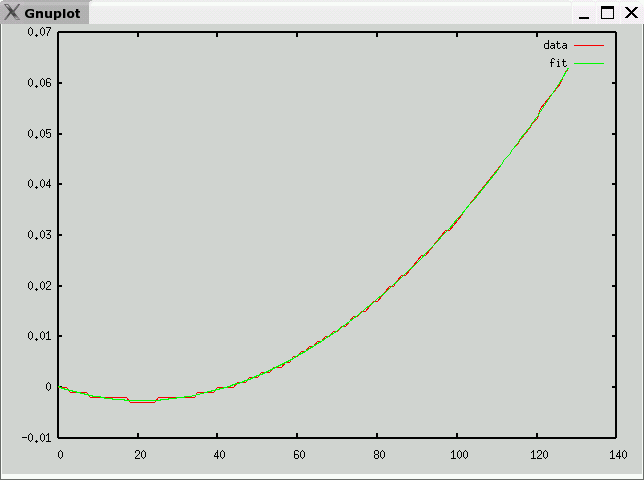# Type B Thermocouple Data and Polynomial

• Description
Pt(30%)-Rh / Pt(60%)-Rh
• Data source
National Institute of Science and Technology (NIST)
• Raw Data
• Octave format
• Curve fit
• mV -> temperature (C)
e is maximum error (in degrees C)
v1 andf v2 partition the domain into three segments, each with a polynomial fit
c is vector of coefficients: a10, a9, ... a0
Note: Each column of c corresponds to one range (<v1,v1 to v2, >v2)

`e = 26.625v1 = 0.43700v2 = 1.8220c =   -3.3455e+09   -1.4991e+02   -1.1016e-08    7.5722e+09   1.6431e+03    1.1361e-06   -7.3495e+09   -7.9377e+03   -5.1819e-05    3.9973e+09   2.2240e+04    1.3848e-03   -1.3362e+09   -4.0005e+04   -2.4161e-02    2.8337e+08   4.8279e+04    2.8985e-01   -3.8027e+07   -3.9658e+04   -2.4484e+00    3.1347e+06   2.2028e+04    1.4762e+01   -1.5123e+05   -8.1042e+03   -6.5404e+01    4.7382e+03   2.1402e+03    3.0364e+02    3.1992e+01   -2.6389e+01   2.0160e+02 `• temperature -> mV (range -127C-127C)
e is maximum error (in mV)
c is vector of coefficients: a10, a9, ... a0

`e = 0.00051429c =    3.4607e-21   -1.5444e-18    2.3360e-16   -6.2362e-15   -2.1839e-12    2.8814e-10   -1.6048e-08    4.4712e-07    1.9541e-07   -2.2761e-04    8.7007e-05 `Previous page: Thermocouples
Next page: Type E